# Molar Equations

## Molar Equations

RECAP:

As you may remember from previous sections, chemical equations are composed of atomic symbols, large numbers and small numbers.

The atomic symbols tell you what atoms are in the chemical reaction.

I.e _Mg + O -> MgO _(This means that 1 atom of magnesium reacts with 1 atom of oxygen to form one molecule of magnesium oxide.)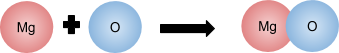The large symbols (in front of atomic symbols) tell you how many molecules of that substance there is in the chemical reaction

I.e 2H + O -> H2O (This means that 2 seperate atoms of Hydrogen react with 1 atom of Oxygen to form one atom of water)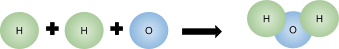The small number tell you how many atoms of a particular type there are in the molecule.

I.e. _2H2 + 2O -> 2H2O _This means that two molecules of hydrogen (each composing of two bonded atoms of hydrogen) reacts with one molecule of Oxygen (made up of two bonded oxygen atoms) forms one molecules of water (composed of two hydrogen atoms and one oxygen atom).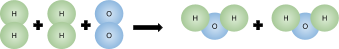Using moles in chemical reactions:

The large numbers (known as stoichiometric numbers) in a chemical equation are VERY useful when dealing with moles of substances. The ratio of moles always stays the same in a chemical reaction. If you are confused by this, just read on, do some examples and it will become very clear!

In the reaction: there is always twice as many moles of Hydrogen and water than there are oxygen. So if you had 3 moles of Oxygen in the reaction, there would be 6 moles of hydrogen reacting with it to form 6 moles of water!

If there was only 1 mole of Oxygen, there would be 2 moles of Water and 2 moles of Hydrogen!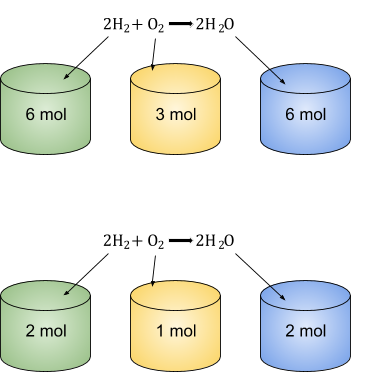Examples:

1. For the chemical reaction:_ 2Mg + O2 -> 2MgO_ how many moles of Magnesium Oxide are produced if there are 5.2 moles of Oxygen reacting.

Answer: The ratio of moles between Oxygen and Magnesium Oxide is 1:2.

There are 5.22 = 10.4 moles of Magnesium Oxide produced.

1. The same chemical reaction takes place but different amounts of substances. If there are now 2 moles of Magnesium reaction, how many moles of Magnesium Oxide are produced?

Answer: The ratio of moles between Magnesium and Magnesium Oxide is 1:1 (since they both have a 2 in front of them!)

There are the same amount of moles of Magnesium and Magnesium Oxide. 2 moles of Magnesium Oxide produced.

TIPS: Make sure to check that the symbol equation is properly balanced. You will get the wrong answers if it is not balanced correctly.

## Limiting Reactions

When two things react, the reaction continues to happen until one (or both) of the reactants have all been used up.

Imagine if you had Carbon reacting with Oxygen in the reaction C + O2 -> CO2. Let’s say that you had a little bit of carbon in a room with lots and lots of Oxygen (the oxygen is in excess). The reaction will continue to happen until one of the reactants has been used up (Carbon runs out before Oxygen since there is lots of Oxygen left). In this example, the Carbon is the limiting reactant.

The amount of product is directly proportional to how much of the limiting reactant you have available.

## Volumes of Gases

At the same temperature and pressure, any 2 gases with the same amount of moles will occupy the same volume of space. So, if you had a box of 1mol Chlorine at 12 degrees and at 100kPa, it would occupy exactly the same volume of space as 1mol Nitrogen gas at 12 degrees and 100kPa!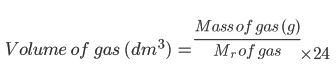## Examples of Molar Equations

Balancing Molar equations using reacting masses

There are two equations which will be your two best friends whilst dealing with molar equations.

whilst dealing with molar equations.

_We have only met one of them so far, and that is: ___Mass (grams) = Mr moles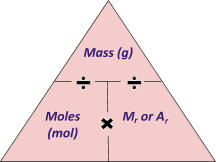If you have the mass of the substances, you can work out the balanced symbol equation for that particular reaction.

__This can be done in 4 steps: __

1. Find the moles of each substance involved, using the equation above. Divide the mass of each substance by its Mr.
2. __Find the ratio of all of the moles of substances. This can be done by dividing each mole by the smallest mole. __
3. If any of the moles are not whole numbers, then multiply them all by the same number to make them whole numbers. (i.e 10.5 mol and 2 mol can be made whole numbers by 2 to make 21 mol and 4 mol.)
4. __Write the balanced symbol equation by putting these numbers in front of the right nuclear symbols. __

__Example 1: __

243g of zinc oxide reacts with 18g of Carbon to form 66g of carbon dioxide and 195g of zinc. Write a balanced symbol equation for this reaction.

__First, calculate the Mr of each substance: __

__ZnO = 65 + 16 = 81 __

__C = 12 __

__CO2= 12 + 16 + 16 = 44 __

Zn = 65

Find how many moles of each substance there are by using the equation above:

__Moles of ZnO = 243/81 = 3mol __

__Moles of C = 18/12 = 1.5mol __

__Moles of CO2 = 66/44 = 1.5mol __

__Moles of Zn = 195/65 = 3mol __

__Now make all of these numbers whole numbers by multiplying by the same thing. It seems easiest to 2. __

__ZnO: 3 mol 2 = 6 mol __

__C: 1.5 mol 2 = 3 mol __

__CO2: 1.5 mol 2 = 3 mol __

__Zn: 3 mol 2 = 6 mol __

Divide each one by the smallest of them. The smallest value is 1.5 mol, so divide each by 1.5:

__ZnO: 3 mol 1.5 mol = 2 __

__ZnO: 1.5 mol 1.5 mol = 1 __

__CO2: 1.5 mol 1.5 mol = 1 __

__Zn: 3 mol 1.5 mol = 2 __

__Now write a balanced equation using these numbers! __

__2ZnO + C CO2 + 2Zn __

__Just double check that you have the same amount of atoms on both sides of the equation. __

Example 2: This is another example with just the maths, some will find example 1 easier to follow, some will find example 2 easier to follow.

672g of Iron reacts with 288g of Oxygen to form 960g of Iron Oxide (Fe2O3). Write a balanced symbol equation for this reaction.

__Mr of each substance is: __

__Fe = 56 __

__O2 = 16 + 16 = 32 __

__Fe2O3= 160 __

__Moles of each substance: __

__Fe: 672g / 56 = 12 mol __

__O2: 288g / 32 = 9 mol __

__Fe2O3: 960g / 160 = 6 mol __

__Divide each by the smallest __

__Fe:12 mol / 6 = 2 __

__O2: 9 mol / 6 =1.5 __

__Fe2O3:6 mol / 6 = 1 __

Multiply all by 2 to make them whole numbers:

__Fe: 2 2 =4 __

__O2: 1.5 2 =3 __

__Fe2O3: 1 2 =2 __

__Write a balanced symbol equation: __

4Fe +3O22Fe2O3

## Examples of Limiting Reactions

When two things react, the reaction continues to happen until one (or both) of the reactants have all been used up.

Imagine if you had Carbon reacting with Oxygen in the reaction C + O2CO2. Let’s say that you had a little bit of carbon in a room with lots and lots of Oxygen (the oxygen is in excess). The reaction will continue to happen until one of the reactants has been used up (Carbon runs out before Oxygen since there is lots of Oxygen left). In this example, the Carbon is the limiting reactant.

The amount of product is directly proportional to how much of the limiting reactant you have available.

## Examples of Volumes of Gases

At the same temperature and pressure, any 2 gases with the same amount of moles will occupy the same volume of space. So, if you had a box of 1mol Chlorine at 12 degrees and at 100kPa, it would occupy exactly the same volume of space as 1mol Nitrogen gas at 12 degrees and 100kPa!

Volume of gas (dm3) =Mass of gas (g)Mr of gas 24